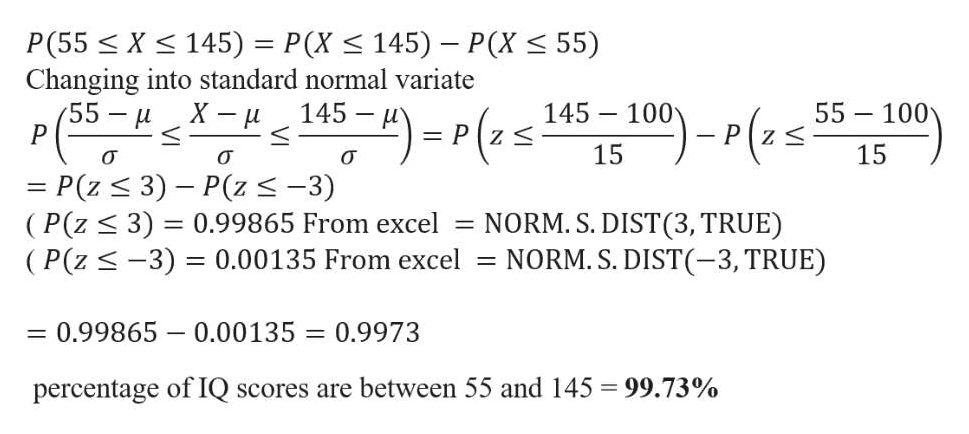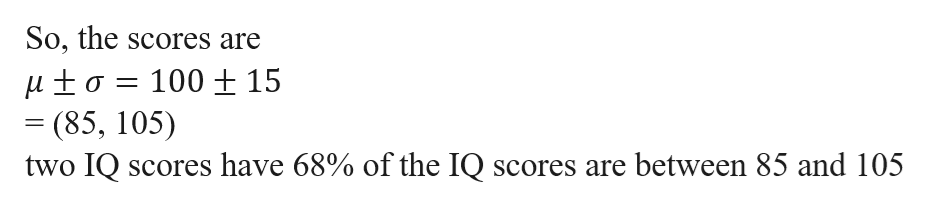# IQ scores have a bell-shaped distribution with a mean of 100 and a standard deviation of 15.a. What percentage of IQ scores are between 55 and 145?b. What two IQ scores have 68% of the IQ scores between them?

Question
16 views

IQ scores have a bell-shaped distribution with a mean of 100 and a standard deviation of 15.

a. What percentage of IQ scores are between 55 and 145?

b. What two IQ scores have 68% of the IQ scores between them?

check_circle

Step 1

Given Data

Mean = 100

Standard Deviation = 15

Step 2

Let X denote the IQ score

a) percentage of IQ scores are between 55 and 145help_outlineImage TranscriptioncloseP(55X145) P(X 145) - P(X Changing into standard normal variate (55 - и P 55) 145 100 45-2) P(1451 10)-(10) 55 100 X — и _ P z s = P(z < - P(z 3) P(z -3) (P(z 3) 0.99865 From excel NORM. S. DIST (3, TRUE) (P(z -3) 0.00135 From excel NORM. S. DIST(-3, TRUE) = 0.99865 - 0.00 135 0.9973 scores are between 55 and 145 99.73% percentage of IQ fullscreen
Step 3

b) two IQ scores have 68% of the IQ scores between are

As per the empirical rule 68% i...help_outlineImage TranscriptioncloseSo, the scores are uto 100 ±15 = (85, 105) two IQ scores have 68% of the IQ scores are between 85 and 105 fullscreen

### Want to see the full answer?

See Solution

#### Want to see this answer and more?

Solutions are written by subject experts who are available 24/7. Questions are typically answered within 1 hour.*

See Solution
*Response times may vary by subject and question.
Tagged in

### Other